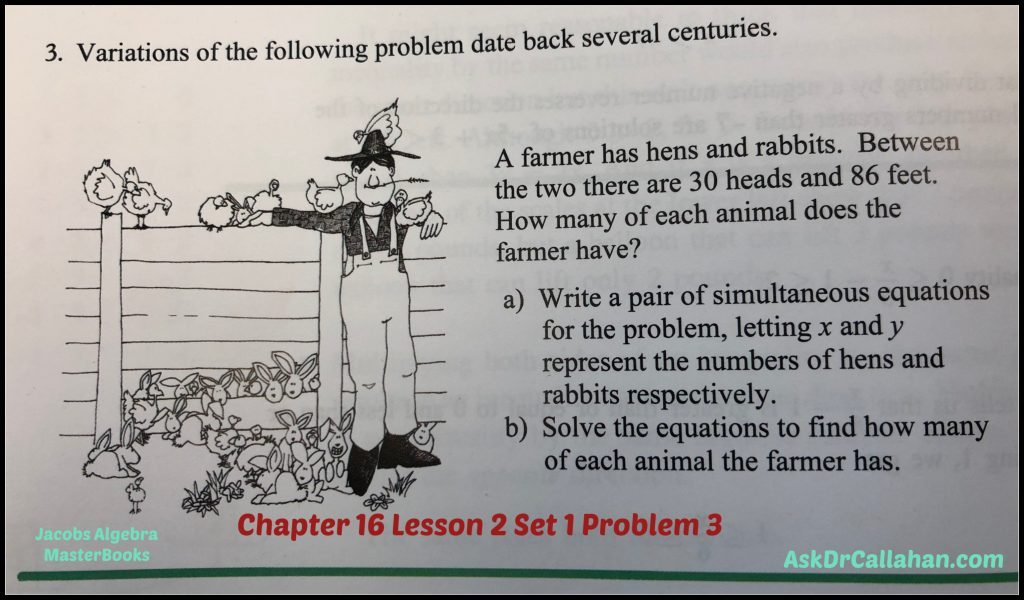Posted on

# Algebra Chapter 16 Lesson 2 Set 1 Problem #3

This is an error in the book by Master Books (ISBN: 978-0890519851) – the book with the blue cover showing the world.

The problem is discussing hens and rabbits with a total of 30 heads and 86 feet.ERROR: The solution manual is referring to the wrong problem which is related to money.

Here is how to solve. Simultaneous equations are covered in Chapter 7. So if you forget how to solve these, refer back to chapter 7.

heads = # hens + # rabbits

feet = 2 x # hens + 4x number of rabbits

So let h be the number of hens and r be the number of rabbits.

We can re-write the above as

heads = 30 = h + r

feet = 86 = 2h + 4r

30=h+r

So r = 30-h

Substitute this equation into the second equation to get

86 = 2h + 4r

86 = 2h + 4(30-h) = 2h + 120 – 4h = -2h +120

Rearrange to get

2h = 120-86 = 34

h = 17 (number of hens)

Now we can find the rabbits are

R = 30-h = 30-17 = 13

Checking our work

13 + 17 = 30 (total number of heads)

2 x 17 + 4 x 13 = 86 which are the number of feet.

Correct!